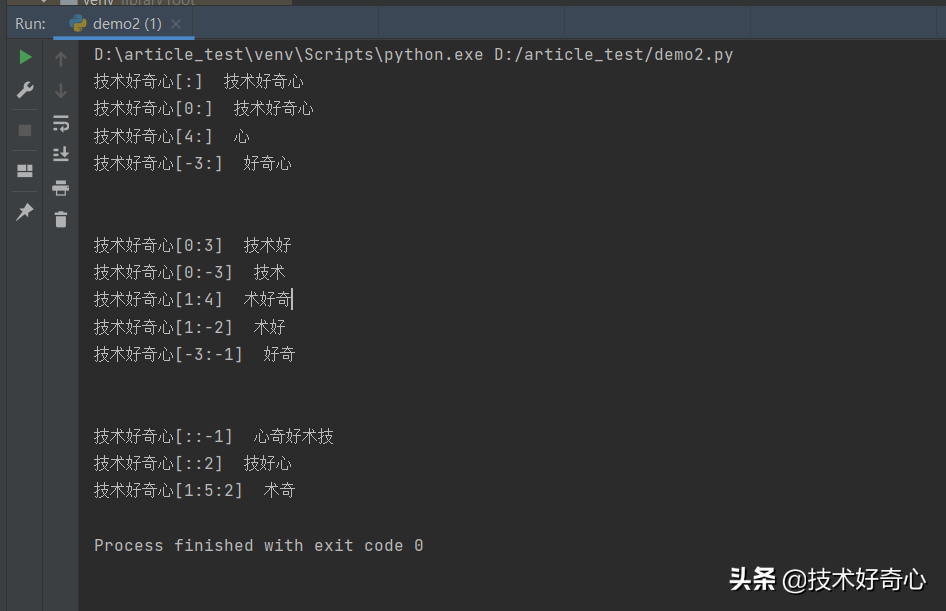# 正常输出文字

``print('技术好奇心，分享技术，一起学习呦^_^')``

``技术好奇心，分享技术，一起学习呦^_^``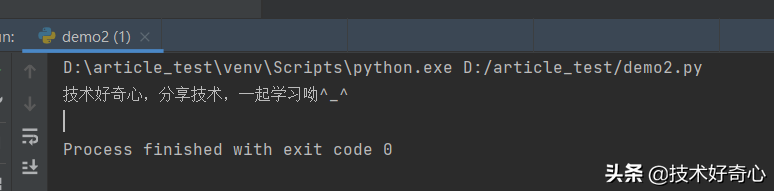# 输出字符串（带单引号或双引号）

``print('技术好奇心，"分享技术，一起学习呦^_^"')print("技术好奇心，'分享技术，一起学习呦^_^'")``

``技术好奇心，"分享技术，一起学习呦^_^"技术好奇心，'分享技术，一起学习呦^_^'``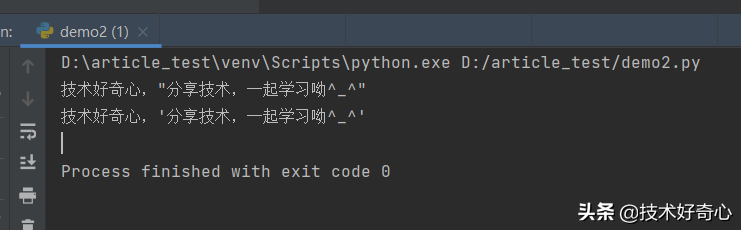# 直接在字符串中换行

``print('''技术好奇心，"分享技术，一起学习呦^_^"''')``

``技术好奇心，"分享技术，一起学习呦^_^"``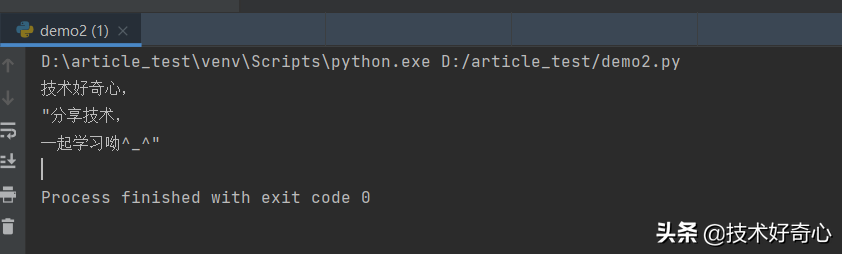# 字符串中使用转义符

• \n：换行
• \t：缩进
• \r：回车

``# 转移特殊符号，比如双引号，让其失去特殊意义，直接显示print("你好，我是技术\"好奇心\"，哈哈哈")# 换行作用print("你好，我是技术好奇心\n，哈哈哈")# tab键，缩进作用print("你好，我是技术好奇心\t，哈哈哈")# 回车键作用print("你好，我是技术好奇心\r，哈哈哈")``

``D:\article_test\venv\Scripts\python.exe D:/article_test/demo2.py你好，我是技术"好奇心"，哈哈哈你好，我是技术好奇心，哈哈哈你好，我是技术好奇心，哈哈哈，哈哈哈``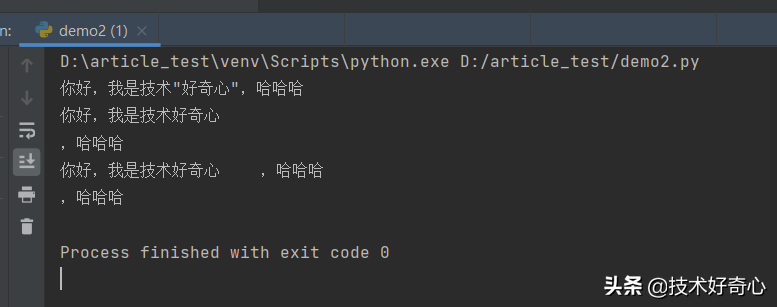# 获取字符串中某个字符

``str = "你好，我是技术好奇心，哈哈哈"print(str)print(str)print(str)print(str)print(str[-1])print(str[-3])``

``D:\article_test\venv\Scripts\python.exe D:/article_test/demo2.py你好，我哈哈``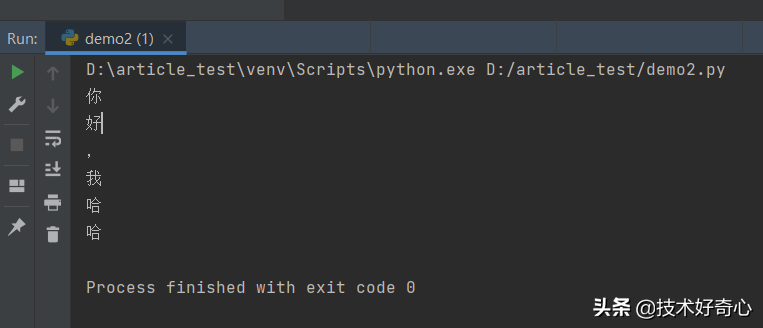# 获取字符串中一段（部分）字符

``str[start : end : step]``
• start：闭区间，包含该下标的字符，第一个字符是 0
• end：开区间，不包含该下标的字符
• step：步长

``# 获取全部字符print("技术好奇心[:] ", '技术好奇心'[:])# 获取全部字符print("技术好奇心[0:] ", '技术好奇心'[0:])# 获取第 5 个字符到最后一个字符print("技术好奇心[4:] ", '技术好奇心'[4:])# 获取倒数第 3 个字符到最后一个字符print("技术好奇心[-3:] ", '技术好奇心'[-3:])print('\n')# 获取第 1 个字符到第 3 个字符print("技术好奇心[0:3] ", '技术好奇心'[0:3])# 获取第 1 个字符直到倒数第 3 个字符print("技术好奇心[0:-3] ", '技术好奇心'[0:-3])# 获取第 2 个字符到第 4 个字符print("技术好奇心[1:4] ", '技术好奇心'[1:4])# 获取第 2 个字符到倒数第 2 个字符print("技术好奇心[1:-2] ", '技术好奇心'[1:-2])# 获取倒数第 3 个字符到倒数第 2 个字符print("技术好奇心[-3:-1] ", '技术好奇心'[-3:-1])print('\n')# 倒序取所有字符print("技术好奇心[::-1] ", '技术好奇心'[::-1])# 步长=2，每两个字符取一次print("技术好奇心[::2] ", '技术好奇心'[::2])# 步长=2，取第 2 个字符到第 5 个字符，每两个字符取一次print("技术好奇心[1:5:2] ", '技术好奇心'[1:5:2])``

``技术好奇心[:]  技术好奇心技术好奇心[0:]  技术好奇心技术好奇心[4:]  心技术好奇心[-3:]  好奇心技术好奇心[0:3]  技术好技术好奇心[0:-3]  技术技术好奇心[1:4]  术好奇技术好奇心[1:-2]  术好技术好奇心[-3:-1]  好奇技术好奇心[::-1]  心奇好术技技术好奇心[::2]  技好心技术好奇心[1:5:2]  术奇Process finished with exit code 0``